# define more fields

We can now define the final fields in Main; Calculation fields whose formulas rely on fields in the related tables. These new fields are listed in the table below.

Field Name

Description

Sav_Current Balance

Current balance of a savings/money market account

Stk_Current Value

Value of a stock based on its current price plus earned dividends

Stk_Change in Value

Change in a stock's value as of the most recent price update

CD_Current Value

Original cost of the CD, plus all interest earned to-date

Before creating the fields, choose FileMaker Pro > Preferences (Mac) or Edit > Preferences (PC), click the Layout tab, uncheck Add newly defined fields to current layout, and click OK. If you leave this option checked, FileMaker automatically places new fields on the layoutfrequently in the worst possible places.

#### Sav_Current Balance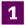Choose File > Define > Database. In the Define Database dialog box, click the Fields tab and select Main from the Table list.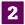Enter Sav_Current Balance in the Field Name box, choose Calculation as the Type, and click Create. The Specify Calculation dialog box appears.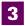Double-click Sav_Initial Balance in the field list on the left. Then type a + (or click the + operator button).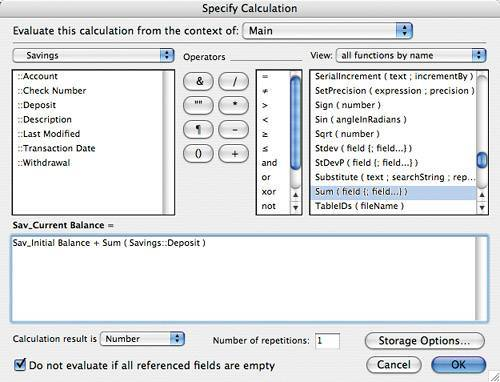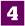Double-click the Sum function in the list on the right. Its arguments are highlighted.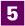Replace the arguments by choosing Savings from the drop-down list above the field list and double-clicking the ::Deposit field. (The :: prefix denotes a related field.)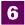Move the cursor to right of the closing parenthesis and then type a - (or click the - operator button).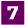Enter another instance of the Sum function by double-clicking it in the function list. Replace the function's arguments by double-clicking the ::Withdrawal field in the field list. The formula should now read:

` Sav_Initial Balance + Sum (Savings::Deposit) - Sum (Savings::Withdrawal) `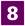To complete the field's definition, make sure the Calculation result is Number, and then click OK.

#### Stk_Current ValueDefine a new Calculation field named Stk_Current Value.In the Specify Calculation dialog box, enter or create the following formula:

`[View full width] (Stk_Current Share Price * Stk_Number of Shares) +Sum (Stocks::Dividend) +Sum (Stocks::Interest ) `

This formula multiplies the current share price by the number of shares held, and then adds in any dividends and interest received (by summing these items from the Stocks table). The components Stocks::Dividend and Stocks::Interest are the Dividend and Interest fields from the Stocks table.To complete the definition, make sure the Calculation result is Number, and then click OK.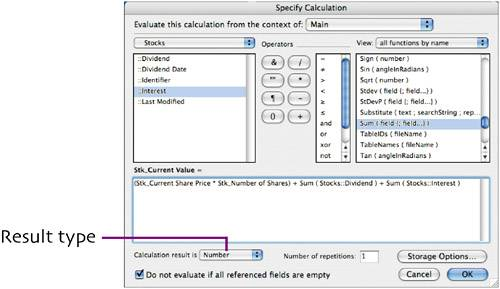The formula is added to the field's definition in the Define Database dialog box.

#### Stk_Change in ValueDefine a new Calculation field named Stk_Change in Value.In the Specify Calculation dialog box, type or create the following formula:

` Stk_Current Value - Stk_Total Cost `

This formula calculates the amount (positive or negative) that the holding has changed by subtracting its original cost (including commission) from the stock's current value, based on the most recent share price recorded. Both fields in the formula are from the Main table.To complete the definition, make sure the Calculation result is Number, and then click OK.

The formula is added to the field's definition in the Define Database dialog box.

#### CD _Current ValueDefine a new Calculation field named CD _Current Value.In the Specify Calculation dialog box, create the following formula:

` CD_Amount + Sum (CD::Interest) `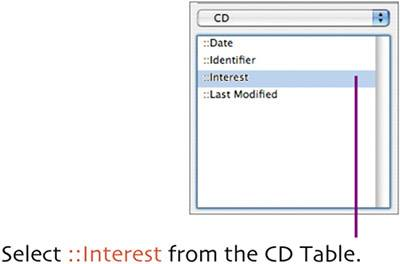The formula computes each CD's value by adding its purchase price to the sum of the accrued interest. (CD_Amount is in the Main table; Interest is in the CD table.)To complete the definition, make sure the Calculation result is Number, and then click OK.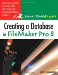Creating a Database in FileMaker Pro 8: Visual QuickProject Guide
ISBN: 0321414837
EAN: 2147483647
Year: 2005
Pages: 115

Similar book on Amazon